# Forces in Skeletal Structure of the Arm

4198 words (17 pages) Essay in Physics

12/03/18

Disclaimer: This work has been submitted by a student. This is not an example of the work produced by our Essay Writing Service. You can view samples of our professional work here.

Any opinions, findings, conclusions or recommendations expressed in this material are those of the authors and do not necessarily reflect the views of UK Essays.

Bio Mechanics and Kinetics

Task 1. Produce a labelled diagram showing the forces involved on the skeletal structure of a human arm as a weight is being held at a particular angle. Your report should use the principle of moments to fully describe all of the forces that are being applied at various points along the forearm through the muscle and tendons. (Assessment criteria 1.1, 1.2, 1.3) Skeletal muscles consist of many parallel fibres wrapped in a flexible sheath along a bone, narrowing at both ends into tendons. Some of the muscles thin into two or three tendons which are known as “biceps” and “triceps”. Muscle fibres contract after receiving an electrical signal from the nerve ending attached to them; hence the muscles shorten and a pulling force is sensed by the two bones. Primarily, the main function of the muscles is to pull and not to push.

As part of this task, I am going to demonstrate the forces involved on the skeletal structure of the human arm when a weight is being held at a particular angle. The image below shows a free-body diagram illustrating the forces exerted on the forearm bar. According to the Laws of Statics such as Newton’s Law, the net force on the immobile bar must be zero, and the total torque (which will be discussed later) is also zero.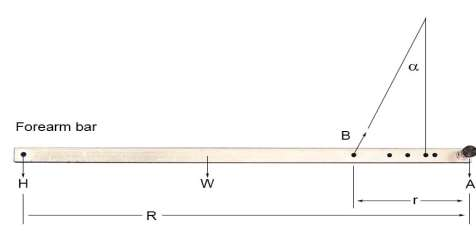Figure 1

Hence the forces acting on the forearm are its weight (W), the weight of the hand (H), force from the bicep muscle (B, which pulls upward the forearm at an angle α) and the force from the humerus bone (A).

The muscular system within the arm generates linear force. Linear force refers to the force that acts in straight line between the origin and the insertion. However the linear force is manifested by the rotational moment which is generated at the joint centre. This is due to the geometrical relationship between the lines of action of the muscles and the joint centre.

The maximum force a muscle is able to exert is equivalent to its cross-sectional area, i.e. the legs are capable of lifting heavier load due to having greater cross-sectional area compared to the arms. Therefore the estimated maximum force a muscle can apply is about 7×106 dyn/cm2 = 7 x 105 Pa = 102lb/in2. The formula to calculate the moment of force is: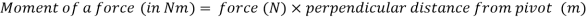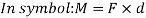For example, if an arm (weighing 7kg) lifts a load of 5kg by 1cm, what is the moment of force applied on the arm?

Firstly I will need to find the force of both objects, by using this formula: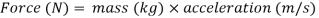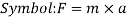Where acceleration is 9.8m/s (Earths gravitational field, since it is constant).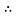The force of the object = 5kg X 9.8m/s = 49N

The force of the arm = 7kg X 9.8m/s = 68.6N

Therefore the moment of a force =49N X 0.01m = 0.49Nm

The moment of force of 0.49Nm is applied on the arm.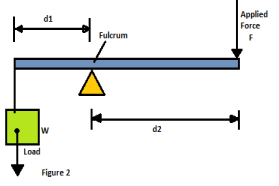The various joints in the body are known as levers which causes rotations about a fulcrum (axis rotation). This is used to figure out the forces exerted by the muscles such as lifting loads and transfer movement from one point to another. For a lever, the force F required to balance a load of weight (W) is:

##### Get help with your essay today, from our professional essay writers!

Qualified writers in the subject of physics are ready and waiting to help you with your studies.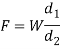Where d1 and d2 are the lengths of the lever arms (illustrated in figure 2)

If d1 is 5cm and d2 is 35cm, find out the force required to balance the weight of 5kg. Using the above formula: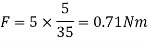Therefore, a force of 0.71Nm is required to balance the weight of 5kg on the arm.

If the load is close to the fulcrum, the mechanical advantage is greater (d12); hence if it is far away then it is smaller (d2> d1). Therefore the mechanical advantage may increase or decrease depending on the distances from the fulcrum.

We can also measure torque (any point of the fulcrum), which refers to the force applied over a distance (lever arm) that causes rotations of the fulcrum. The torque is dependent on three variables: amount of force, angle of application of force and the length of the moment arm/ R. As mentioned above in figure 1, the total torque is equal to zero;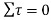. The following formula is used to calculate Torque τ: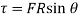Where F is the force (0.71Nm),

R is the distance from the location force is applied to the joint (moment arm) (35cm)

Ï´ is the angle between the force and the radial line

I will now find out the torque for the same question, if the angle is 20°;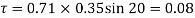This links in with the above statement of the total torque being equal to zero.

I am now going to discuss about the elbow and the forces applied to it. There are many properties which can be used to calculate the forces of the biceps: the angle of the elbow; the length of the upper and lower arm bone; and the distance from the bone to the location the muscle is attached to.

I will now use this formula to find out the force exerted by the biceps (equilibrium) in holding the object, which is the sum of the clockwise moments about any points, equals the sum of the anticlockwise moments about the same point: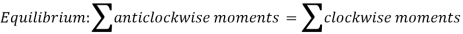Taking 5cm from bone to the biceps attachment;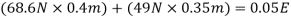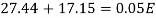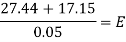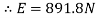The force exerted by the biceps holding the object is 891.8N.

Similarly, we can also measure the tension of the bicep/arm holding the object. The image below shows an arm being held out and elevated from the shoulder by the deltoid muscle. The forces can be measured the taking the sum of the torques (of the shoulder joint, the tension (T) can be calculated: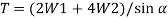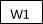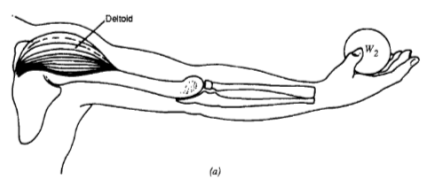Where W1 is the weight of the arm,

W 2 is the weight of the object

Using the above question; if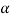= 20; the weight of the arm (W1) is 68.6N and the weight of the object (W2) is 49N, then calculate T: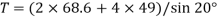= 113.96N

Therefore the force needed to hold up the bicep/arm at 20, is 113.96N.

Task 2. A) You must complete the energy changes/momentum worksheet. Assessment criteria 2.3,2.4

See attachments

b) You must produce a report that describes the equations of motion needed to calculate the range and maximum height that a projectile thrown by a human can achieve. This report must include examples of both the range equation and maximum height equation. You could use a sports person throwing a ball as an example.

A projectile is any object that has been thrown or shot by a human (measures projectile motion). Projectiles are affected by two factors: gravity (Horizontal motion) and air resistance (vertical motion which is the force of gravity pulling down the object).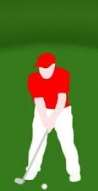As part of this task I am going to carry out various calculations to find out the range and maximum height that a golf ball can achieve when a golf player hits the ball.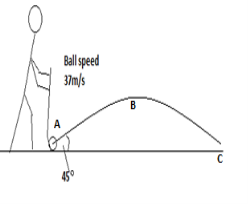A golfer hits a ball so that it moves off with a speed of 37m/s at an angle of 45. I am going to calculate how far the ball goes; the maximum height it will reach; and how long it takes for the ball to get there.

Firstly, I am going to use the following formula to calculate how far the ball travels;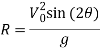Where R is the range/resultant (how far the ball goes),

V0 is the initial velocity of the ball speed (37m/s)

g is the gravity (9.8m/s) also can be used as (a) since it is constant

Ï´ is angle of the ball (45°)

Therefore;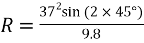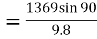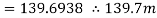Hence, when a ball is hit with a speed of 37m/s at 45°, the ball will go far as 139.7m.

##### Get help with your essay today, from our professional essay writers!

Qualified writers in the subject of physics are ready and waiting to help you with your studies.

Secondly, I will calculate the maximum projectile height (how high a ball will go) by using the following method;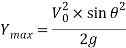Where Ymax is the maximum projectile height that the ball will go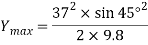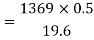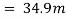The maximum projectile height that a ball will reach is 34.9m.

The final calculation that I am going to carry out is the flight time so that I can find out how long it takes for the ball to get there. I will use the following method;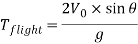Where Tflight is the time flight of how long it takes for the ball to reach there.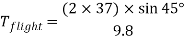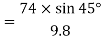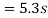The flight time for the ball to get there is 5.3s.

Using the same question, I now want to find out how far the ball travels horizontally from A to C and the time that the ball is in the air, ignoring any air resistance and taking g = 10ms-2.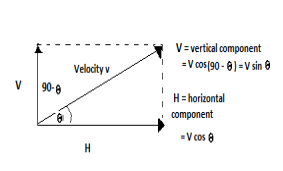Firstly, I will calculate the time that the ball is in the air for, by using the following formula;

I need to find out the vertical motion from A to B first = 90° – 45° = 45°

Formula;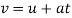Where v is the final velocity (0 since it is moving horizontally),

u is the initial velocity (37m/s x cos 45) is 26.16m/s

a is the acceleration (10m/s)

t is the time

Therefore;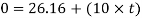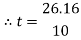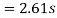, so the time it takes from A to C is twice this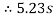I will now look at the horizontal motion from A to C.

Horizontal component of velocity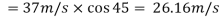. This is constant during motion.

Horizontal distance = horizontal velocity X time of flight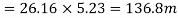Therefore the horizontal distance the ball travels from A to C is 136.8m.

Task 3. You must produce a report showing how the variation of blood pressure affects the human body. Your report must include calculations to determine pressure based on area or density values. Assessment criteria 3.1,3.2

Bernoulli’s Principles explains that flowing blood has different speeds and therefore different kinetic energies (KE) at different parts of the arteries. It determines the relationships between the pressure, density and velocity at every point in a fluid. Bernoulli’s Principle was discovered by a Swiss physicist called Daniel Bernoulli in 1738. He has demonstrated that as the velocity of fluid flow increases, its pressure decreases.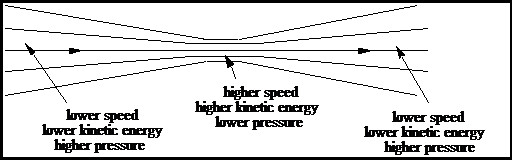Flowing blood has mass and velocity. The mean velocity squared (V2) is equal to the kinetic energy. The image below demonstrates the variance of kinetic energy at different parts of the vessels and also shows the theory of Bernoulli’s Principle:

Therefore KE = ½ mV2. As we know from above that blood flows inside arteries, were pressure is applied laterally against the walls of the vessel which is known as the potential or pressure energy (PE). The total energy (E) of the blood pressure within the artery is the sum of the kinetic and potential energies (presuming there are no gravitational effects):

E = KE + PE(where KE ∝ V2)â€ƒ Therefore,E ∝ V2 + PE

Similarly, Bernoulli’s Principle states that the sum of the Pressure (P), the kinetic energy per unit volume (1/2 pv2), and the gravitational potential energy per unit volume (pgy) has the same value at all points along a streamline. The equation below shows this: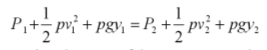There are two vital theories that follow from this relationship, which includes:

• Blood flow driven by the variation in total energy between two points. Normally, pressure is considered as the driving force for blood flow but in fact it is the total energy that moves flow between two areas (i.e. longitudinally along a blood vessel or across a heart valve). KE is relatively low in most of the cardiovascular system; hence PE difference is the energy that drives flow. Similarly, is KE is high then the total energy increases which explains the flow across the aortic valve during cardiac ejection. This is because, as KE drives blood across the valve at a very high velocity, it ensures that the total energy (E) in the blood crossing the valve is higher than the total energy of the blood more distal in the aorta.
• KE and PE can be converted to maintain the total energy unchanged, which is the basis of Bernoulli’s Principle. This principle is basically about the blood vessel that is suddenly narrowed then returned to its normal diameter. The velocity increases as the diameter decreases in narrowed region (stenosis). Blood flow (F) is the mean velocity (V) and the vessel cross-sectional area (A) is directly related to diameter (D) (or radius, r2); hence V ∝ 1/D2. If the diameter is reduced by half in the region of the stenosis, the velocity increases 4-fold, due to KE ∝ V2, hence KE increases 16-fold. The image below demonstrates this: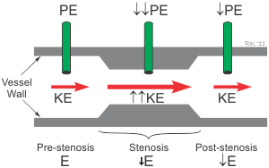The image above shows the total energy being conserved within the stenosis (E actually decreases because of resistance), then the 16-fold increase in KE will decrease in PE. Once past the narrowed segment, KE will go back to its pre-stenosis value as the post-stenosis diameter is the equal to the pre-stenosis diameter, hence flow is conserved. Due to the resistance of the stenosis and turbulence, the port stenosis PE and E will both fall. Therefore, blood flowing at greater velocities has greater ratio of KE to PE.

As we know, blood pressure is the force of fluid against the walls of the arteries, similar to how water exerts the pressure inside aplastic pipe. It is made up of systolic and diastolic pressure. Systolic pressure is the top figure and relates to when the heart is contracting (beating) and forcing blood through the arteries and transporting it to the rest of the body i.e. brain, kidneys etc. The normal values for systolic pressure is from 120-139mmHg. Diastolic pressure is the bottom figure and is linked to when the heart is relaxing. The normal value for diastolic pressure is between 80-89mmHg. Hence, if the values exceed these numbers, then it is considered to be hypertension.

##### Get help with your essay today, from our professional essay writers!

Qualified writers in the subject of physics are ready and waiting to help you with your studies.

Hypertension is high blood pressure which is caused by the increased force of blood flowing through the arteries. Healthy arteries are flexible, strong and elastic. Inner lining of the arteries are smooth allowing the blood to flow freely, supplying important organs and tissues with adequate nutrients andoxygen. Hypertension can gradually lead to wide range of problems such as damaging the cell’s inner lining of the arteries; hence releasing a cascade of events that causes the artery wallsthick and stiff (called arteriosclerosis), or hardening of the arteries. Similarly, the fats from the diet enter the bloodstream and passes through the damaged cells. However, plaques are prone to building up in the arteries leading to atherosclerosis. These changes lead to blocking blood flow to the heart, kidneys, brain, arms and leg.

• Heart: it causes coronary artery disease, which is narrowing of the arteries that doesn’t allow blood to flow freely through the arteries. Leading to chest pain, heart attack (myocardial infarction)or irregular heart rhythms (arrhythmias). Hypertension can also cause enlarged left heart as the pressure forces the heart to work harder than necessary. Similarly, overtime the strain on the heart leads to the heart muscles to weaken and work less effectively causing the heart to wear out and fail.
• Brain: – hypertension can cause mini-strokes (Transient ischemic attack (TIA)), which a temporary disruption of blood supply to the brain caused by blood clot or atherosclerosis. Similarly, it can lead to the full-blown stroke which happens when part of the brain is deprived of oxygen and nutrients leading brain cells to die. Uncontrolled hypertension damages and weakens the brain’s blood vessels, causing to narrow, rupture or leak. Narrowing and blockage of the brain’s blood vessels can also lead to Dementia and mild cognitive impairment.
• Kidneys: – filters excess fluid and waste from the blood via a process that depends on healthy blood vessels. Hypertension can damage both the blood vessels and leading to the kidneys. This leads to various kidney diseases, failure and scarring of the kidney.
• Eyes:- Blood vessels supplies blood to the eyes; hence high blood pressure can damage the blood vessel (retinopathy)

Reference http://www.bhf.org.uk/heart-health/conditions/high-blood-pressure/blood-pressure-research.aspx 22nd October 2013

http://www.cvphysiology.com/Hemodynamics/H012.htm 26th October 2013

http://www.bu.edu/gk12/kai/Lesson%206/BP_Back.pdf 27th October 2013

http://mysite.verizon.net/fvozzo/genphys/lecture.html 27th October 2013

http://muscle.ucsd.edu/musintro/ma.shtml 29th October 2013

http://www.as.wvu.edu/~rbrundage/chapter8b/sld015.htm 29th October 2013

http://www.answers.com/topic/projectile-motion 30th October 2013

http://demoweb.physics.ucla.edu/node/28 25th November 2013

http://www.medicalphysics.org/apps/medicalphysicsedit/WebPOTB.pdf 25th November 2013

http://physics.eou.edu/opensource/physics/projectile.pdf 27th November 2013

Dobson et al. (2002). ‘ Collin advanced – Physics’. Collins eduction : London

Rounce, J.F and Lowe, T.L. (1992). ‘Calculations for A level Physic’. Second edition. Stanley Thornes: Britain

Boutal et al. (2008). ‘AS-Level physics – exam board’. Coordination group publications CGP: Newcastle

Tsokos, KA. (2008). ‘Physics for the IB Diploma’. Fifth edition. Cambridge university press: united kingdom

Johnson et al. (2000). ‘Advanced physics for you’. Nelson Thornes: united kingdom

#### Cite This Work

To export a reference to this article please select a referencing stye below:

## Related Services

View all

### DMCA / Removal Request

If you are the original writer of this essay and no longer wish to have the essay published on the UK Essays website then please.Prices from
###### £28

Undergraduate 2:2 • 250 words • 7 day delivery

Delivered on-time or your money back

##### Rated 4.6 out of 5 by(87 Reviews)